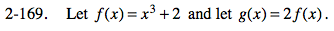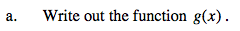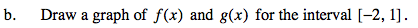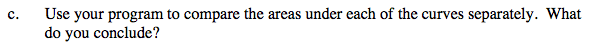### Home > PC > Chapter 2 > Lesson 2.3.8 > Problem2-169

2-169.
1. Let f(x) = x3 + 2 and let g(x) = 2 f(x). Try this using the 2-169 eTool (Desmos). Homework Help ✎

1. Write out the function g(x).

2. Draw a graph of f(x) and g(x) for the interval [−2, 1].

3. Use your program to compare the areas under each of the curves separately. What do you conclude?Use the eTool below.
Click the link at right for the full version of the eTool: PCT 2-169 HW eToolf(x) = 2x3 + 4